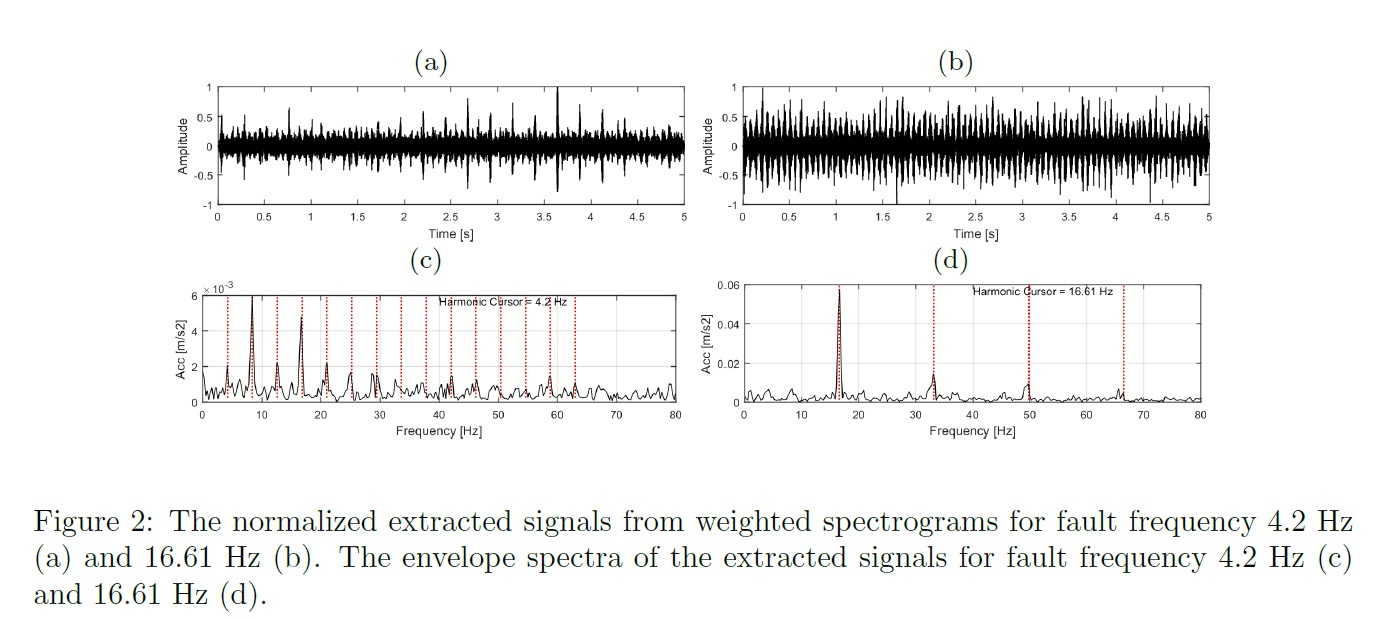# Local damage detection related to a gearbox operating in industrial conditionsThe problem of local damage detection is widely discussed in the literature. There are many methods which can be applied, however there is still a need for new techniques addressing specific diagnostic issues. In particular, the case of complex multiple-component vibration signal is a challenging problem. In this case we focus on such a problem related to a gearbox operating in industrial conditions.

The proposed methodology consists of six main steps. In the first one the vibration signal$x(t)$ is converted into the time-frequency domain. We propose to use the spectrogram, which is based on the short-time Fourier transform. The formula for spectrogram is following:${\rm\bf Spec(t,f)}=\left|STFT(t,f)\right|^2=\left|\sum_{m=0}^{K-1}w(t-m)x(m)e^{-2i\pi fm/K}\right|^2,$
where$w(\cdot)$ is a$W$-long window function,$t=1,\ldots,N$ is a time point,$f$ is a frequency bin and$K\geq M$ is the number of points in which the Fourier Transform is calculated. Given the time-frequency representation of the data, time series related to each frequency bin are examined for having cyclic properties. Periodic amplitude modulation, shared by several frequency bins, might be a signature of local damage. It starts with spectrogram-based decomposition of the signal on subbands with following parameters:$N_w$ – window length,$Ov$ – overlap (percentage of overlapping windows),$fs$ – sampling frequency and$NFFT$ – the number of FFT points. In the next step the analyzed fault frequency has to be set.

In the following step for each frequency bin in the time series${\rm\bf Spec}(:,f)$ local maxima are found. The crucial parameter in this step is the range in which the maximum is founded. Low range leads to large number of local maxima unrelated to local damage. On the other hand, wide range could result in some significant fault signatures omitted. Therefore, we propose to relate the range with considered fault frequency, i.e.$r=\left\lfloor \frac{2}{3}\frac{fs}{f_f N_w(1-O_v)}\right\rfloor$. Such$r$ translates the period related to fault frequency into the spectrogram time axis. The factor$\frac{2}{3}$ is responsible for slight reduction of the range, since subsequent fault-related local maxima might occur on the boundary of the period$1/f_f$. The binary function that indicates if the time point$t_i$ reveals the local maximum might be defined as:$M(t_i,f)= \begin{cases} 1, \;\;\;\text{if}\; {\rm\bf Spec(t,f)}(t_i,f)=\max_{i-r\leq k \leq i+r}{{\rm\bf Spec(t,f)}(t_k,f)} \\ 0, \;\;\;\text{otherwise}.\\ \end{cases}$

Thereafter each subband in$M$ is considered separately. Given$f$, a score, which quantifies periodicity, is assigned to each$t_i$. It evaluates the average of$M$ values at time points$(\ldots,t_{i}-2T,t_{i}-T,t_i,t_{i}+T,t_{i}+2T,\ldots)$, namely$score(t_i,f)=\frac{\sum_{1 \leq t_i + kT \leq \lfloor N/T\rfloor}M(t_i+kT,f)}{\lfloor N/T\rfloor},$ where$T=\left\lfloor \frac{fs}{f_f N_w(1-O_v)}\right\rfloor$ is the fault-related period (in samples) and$k\in \mathbb{Z}$. The score matrix indicates the average number of local maxima in time points spaced by$T$. Thus, it might be considered as a time-varying filter with periodic coefficients, since$score(t_i,f)=score(t_i + kT,f)$. In order to return to the time domain the STFT is multiplied element-wise by the score matrix. Then, the inverse short-time Fourier transform algorithm is applied to such STFT with modified amplitudes and filtered signal $y(t)$ might be further analyzed:$y(t)=\int_{-\infty}^\infty\int_{-\infty}^\infty STFT_x(t,f)score(t,f)e^{2\pi i ft}dt df.$

As the result both the filtered and raw signals are of the same length. Amplitude of$y(t)$ reflects not only presence of periodic local maxima in raw signal spectrogram but also real amplitudes related to them. Thus, the proposed method takes into account presence of periodic amplitude modulation in each subband separately, and the corresponding energy. It is worth mentioning that in case of real signal, which is cyclostationary of order 2 (CS2),$y(t)$ is also CS2. Therefore, the standard envelope based method can be applied. On the other hand, proposed filtration provide the signal, which contain only the cyclic components, thus the proper harmonics should be more visible in the envelope spectrum.

The results of the methodology application to the vibration data recorded on the belt conveyor gearbox are presented. Analyzed machine is located in the underground copper ore mine, thus it works in harsh environment.

The signal reveals two faults in the machine related to the rotating speed of first (16.61 Hz) and second shaft (4.2 Hz). Score maps for two fault frequencies are presented in Figs. 1a and 1b. Some barely visible periodic patterns might be noticed in both figures. The pattern related to 4.2 Hz is located in carrier frequency band lower than 1000 Hz. The second pattern reveals in almost every frequency band, including the lowest frequencies.Weighted spectrograms are illustrated in Figs. 1c and 1d. One can observe that the level of background noise is relatively small and the periodic excitations are with relatively high amplitudes. In order to transform weighted spectrogram to time domain the inverse short-time Fourier transform can be applied. Therefore, the cyclic component is extracted. The results are presented in Figs. 2a and 2b. Two pulse trains with modulation frequency 4.2 Hz might not be clearly visible, since the corresponding score values are relatively low. Excitations related to the second fault are clearly visible. Given the extracted components, envelope analysis might be performed in order to check periodicity of the extracted signals. According to Figs. 2c more than 10 harmonics of 4.2 Hz might be noticed which corresponds to impulsive character of the source signal. It is worth to notice that in the carrier frequency band 0-1000 Hz both modulation frequencies are revealed. Moreover, filter coefficients related to 16.61 Hz are the highest in the band from 0 Hz up to 2000 Hz, among both filter characteristics – it ensures that there are significant local maxima with related range. Nevertheless, the signal filtered using the weighted spectrogram calculated for 4.2 Hz does not contain significant amplitude modulations of 16.61 Hz. Hence, even such sources might be separated and damage detection can be performed with introduced novel method.

By Piotr Kruczek, PhD student, Faculty of Pure and Applied Mathematics, Wrocław University of Science and Technology